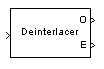Documentation

# Deinterlacer

Distribute elements of input vector alternately between two output vectors

## Library

Sequence Operations## Description

The Deinterlacer block accepts an even length column vector input signal. The block alternately places the elements in each of two output vectors. As a result, each output vector size is half the input vector size. The output vectors have the same complexity and sample time of the input.

This block accepts a column vector input signal with an even integer length. The block supports the following data types: `int8`, `uint8`, `int16`, `uint16`, `int32`, `uint32`, `boolean`, `single`, `double`, and fixed-point. The output signal inherits its data type from the input signal.

The Deinterlacer block can be useful for separating in-phase and quadrature information from a single vector into separate vectors.

## Examples

If the input vector has the values [1; 5; 2; 6; 3; 7; 4; 8], then the two output vectors are [1; 2; 3; 4] and [5; 6; 7; 8]. Notice that this example is the inverse of the example on the reference page for the Interlacer block.

If the input vector has the values [1; 2; 3; 4; 5; 6], then the two output vectors are [1; 3; 5] and [2; 4; 6].

Interlacer